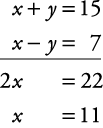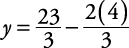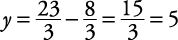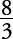## Number Problems with Two Variables

Here are some examples for solving number problems with two variables.

##### Example 1

The sum of two numbers is 15. The difference of the same two numbers is 7. What are the two numbers?

First, circle what you're looking for— the two numbers. Let x stand for the larger number and y stand for the second number. Now, set up two equations.

The sum of the two numbers is 15.

x + y = 15

The difference is 7.

xy = 7

Now, solve by adding the two equations.Now, plugging into the first equation givesThe numbers are 11 and 4.

##### Example 2

The sum of twice one number and three times another number is 23 and their product is 20. Find the numbers.

First, circle what you must find— the numbers. Let x stand for the number that is being multiplied by 2 and y stand for the number being multiplied by 3.

Now set up two equations.

The sum of twice a number and three times another number is 23.

2 x + 3 y = 23

Their product is 20.

x( y) = 20

Rearranging the first equation gives

3 y = 23 – 2 x

Dividing each side of the equation by 3 givesNow, substituting the first equation into the second givesMultiplying each side of the equation by 3 gives

23 x – 2 x 2 = 60

Rewriting this equation in standard quadratic form gives

2 x 2 – 23 x + 60 = 0

Solving this quadratic equation using factoring gives

(2 x – 15)( x – 4) = 0

Setting each factor equal to 0 and solving givesWith each x value we can find its corresponding y value.

If, thenor.

If x = 4, thenor.

Therefore, this problem has two sets of solutions.

The number being multiplied by 2 is, and the number being multiplied by 3 is, or the number being multiplied by 2 is 4 and the number being multiplied by 3 is 5.